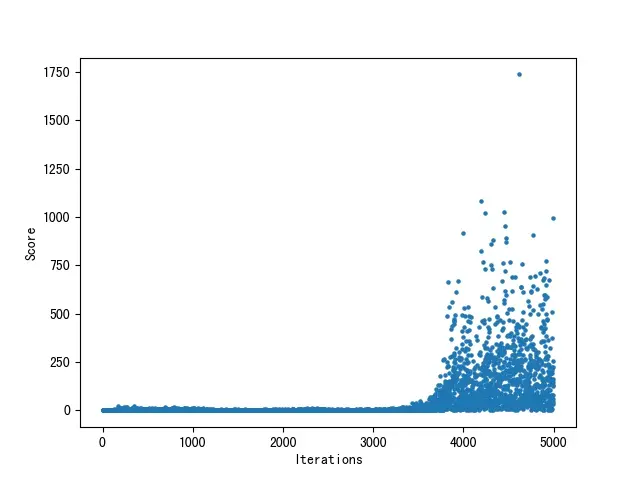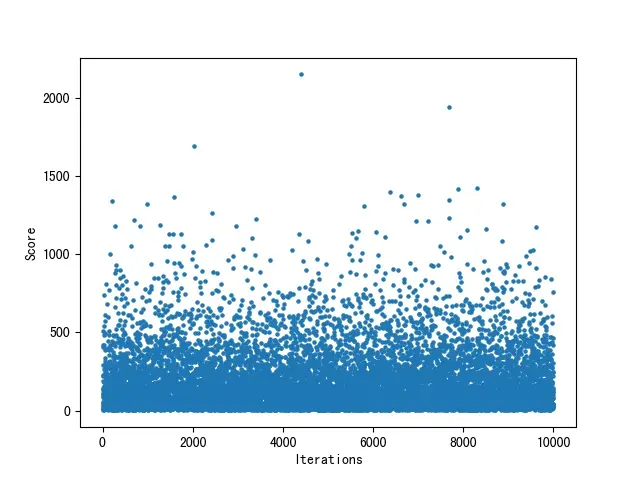## Flappy Bird

Flappy Bird was a side-scrolling mobile game, the objective was to direct a flying bird, named “Faby”, who moves continuously to the right, between sets of Mario-like pipes. Note that the pipes always have the same gap between them and there is no end to the running track. If the player touches the pipes, they lose. Faby briefly flaps upward each time that the player taps the screen; if the screen is not tapped, Faby falls because of gravity; each pair of pipes that he navigates between earns the player a single point, with medals awarded for the score at the end of the game. Android devices enabled the access of world leaderboards, through Google Play. You can also play this game on-line: http://flappybird.io/.

## Tasks

Implement the algorithm in the tutorial example , and output the Q-matrix and the path with the highest values.

Now here is a flappy bird project (Python3) for you, and the file bot.py is incomplete. You should implement a flappy bird bot who learns from each game played via Q-learning.

Please pay attention to the following points:

• The state of the bird is defined by the horizontal and vertical distances fro the next pip and the velocity of the bird.
• In order to understand the state space, you have You need to briefly understand the following sizes: SCREENWIDTH=288,SCREENHEIGHT=512, PIPEGAPSIZE=100, BASEY=SCREENHEIGHT*0.79, PIPE=[52,320],PLAYER=[34,24],BASE=[336,112], BACKGROUND=[288,512],etc.
• The Q values are dumped to the local JSON file qvalues.json.
• initialize_qvalues.py is an independent file, and we can run python initialize_qvalues.py to initialize the Q values. Of course, this file has been initialized.
• You can run python learn.py --verbose 5000 to update the Q values dumped to qvalues.json with 5000 iterations, and then run python flappy.py to observe the performance the bird.

Please complete the function update_scores() in bot.py, and run python learn.py --verbose 5000 and python learn.py --verbose 10000 to get the following figures, respectively.

## Codes and Results

### QLearning.py

import numpy

def qLearning(q, r, gamma=0.8, epsilon=0.4, max_iter=100, end=5):
for _episode in range(max_iter):
state = numpy.random.randint(0, 6)
while state != end:
possible_actions = []
possible_q = []
for action in range(6):
if r[state, action] >= 0:
possible_actions.append(action)
possible_q.append(q[state, action])

action = -1
if numpy.random.random() < epsilon:
action = possible_actions[numpy.random.randint(
0, len(possible_actions))]
else:
action = possible_actions[numpy.argmax(possible_q)]

q[state, action] = r[state, action] + gamma * q[action].max()

state = action
return q

def getPath(q, beg=2, end=5):
state = beg
path = [state]
while state != end:
state = q[state].argmax()
path.append(state)
return path

if __name__ == "__main__":
q = qLearning(q=numpy.matrix(numpy.zeros([6, 6])), r=numpy.matrix([
[-1, -1, -1, -1,  0,  -1],
[-1, -1, -1,  0, -1, 100],
[-1, -1, -1,  0, -1,  -1],
[-1,  0,  0, -1,  0,  -1],
[0, -1, -1,  0, -1, 100],
[-1,  0, -1, -1,  0, 100]]))
print(q)
print(getPath(q))


[[  0.    0.    0.    0.   80.    0. ]
[  0.    0.    0.   64.    0.  100. ]
[  0.    0.    0.   64.    0.    0. ]
[  0.   80.   51.2   0.   80.    0. ]
[ 64.    0.    0.   64.    0.  100. ]
[  0.    0.    0.    0.    0.    0. ]]
[2, 3, 1, 5]


### bot.py

import json

class Bot(object):
"""
The Bot class that applies the Qlearning logic to Flappy bird game
After every iteration (iteration = 1 game that ends with the bird dying) updates Q values
After every DUMPING_N iterations, dumps the Q values to the local JSON file
"""

def __init__(self):
self.gameCNT = 0  # Game count of current run, incremented after every death
self.DUMPING_N = 25  # Number of iterations to dump Q values to JSON after
self.discount = 1.0
self.r = {0: 1, 1: -1000}  # Reward function
self.lr = 0.7
self.load_qvalues()
self.last_state = "420_240_0"
self.last_action = 0
self.moves = []

def load_qvalues(self):
"""
Load q values from a JSON file
"""
self.qvalues = {}
try:
fil = open("qvalues.json", "r")
except IOError:
return
self.qvalues = json.load(fil)
fil.close()

def act(self, xdif, ydif, vel):
"""
Chooses the best action with respect to the current state - Chooses 0 (don't flap) to tie-break
"""
state = self.map_state(xdif, ydif, vel)

self.moves.append(
(self.last_state, self.last_action, state)
)  # Add the experience to the history

self.last_state = state  # Update the last_state with the current state

if self.qvalues[state] >= self.qvalues[state]:
self.last_action = 0
return 0
else:
self.last_action = 1
return 1

def update_scores(self, dump_qvalues=True):
"""
Update qvalues via iterating over experiences
"""
history = list(reversed(self.moves))

# Flag if the bird died in the top pipe
'''
high_death_flag = True if int(
history.split("_")) > 120 else False
'''
# Q-learning score updates
# Your code here
for t, (state, act, next_state) in enumerate(history):
# Select reward
# Your code here
if t < 3:
now_reward = self.r + t * 50
else:
now_reward = self.r

# Update self.qvalues[state][act]
# Your code here
self.qvalues[state][act] = (1 - self.lr) * self.qvalues[state][act] + self.lr * (
now_reward + self.discount * max(self.qvalues[next_state]))

self.gameCNT += 1  # increase game count
if dump_qvalues:
self.dump_qvalues()  # Dump q values (if game count % DUMPING_N == 0)
self.moves = []  # clear history after updating strategies

def map_state(self, xdif, ydif, vel):
"""
Map the (xdif, ydif, vel) to the respective state, with regards to the grids
The state is a string, "xdif_ydif_vel"

X -> [-40,-30...120] U [140, 210 ... 420]
Y -> [-300, -290 ... 160] U [180, 240 ... 420]
"""
if xdif < 140:
xdif = int(xdif) - (int(xdif) % 10)
else:
xdif = int(xdif) - (int(xdif) % 70)

if ydif < 180:
ydif = int(ydif) - (int(ydif) % 10)
else:
ydif = int(ydif) - (int(ydif) % 60)

return str(int(xdif)) + "_" + str(int(ydif)) + "_" + str(vel)

def dump_qvalues(self, force=False):
"""
Dump the qvalues to the JSON file
"""
if self.gameCNT % self.DUMPING_N == 0 or force:
fil = open("qvalues.json", "w")
json.dump(self.qvalues, fil)
fil.close()
print("Q-values updated on local file.")

python learn.py --verbose 5000python learn.py --verbose 10000